Courses

# Test: Surds And Indices- 2

## 10 Questions MCQ Test Quantitative Aptitude for Banking Preparation | Test: Surds And Indices- 2

Description
This mock test of Test: Surds And Indices- 2 for Quant helps you for every Quant entrance exam. This contains 10 Multiple Choice Questions for Quant Test: Surds And Indices- 2 (mcq) to study with solutions a complete question bank. The solved questions answers in this Test: Surds And Indices- 2 quiz give you a good mix of easy questions and tough questions. Quant students definitely take this Test: Surds And Indices- 2 exercise for a better result in the exam. You can find other Test: Surds And Indices- 2 extra questions, long questions & short questions for Quant on EduRev as well by searching above.
QUESTION: 1

### (132)7 × (132)? = (132)11.5.

Solution: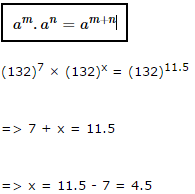QUESTION: 2

###Solution: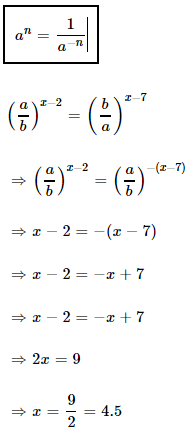QUESTION: 3

### If 7(x - y) = 343 and 7(x + y) = 16807, what is the value of x?

Solution: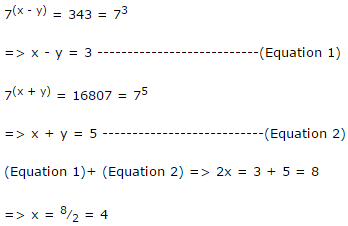QUESTION: 4

(0.04)-2.5 = ?

Solution: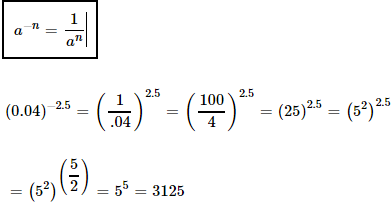QUESTION: 5

(6)6.5 × (36)4.5 ÷ (216)4.5 = (6)?

Solution:

(6)6.5 × (36)4.5 ÷ (216)4.5

= (6)6.5 × [(6)2]4.5 ÷ [(6)3]4.5

= (6)6.5 × (6)9 ÷ (6)13.5

= (6)(6.5 + 9 - 13.5)

= (6)2

QUESTION: 6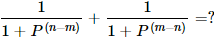Solution: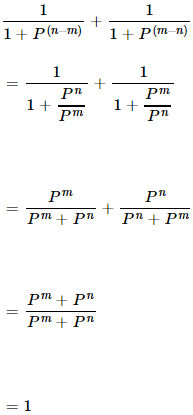QUESTION: 7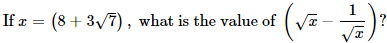Solution: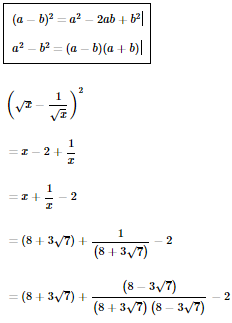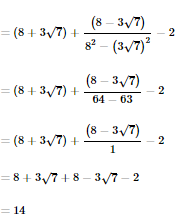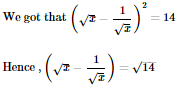QUESTION: 8

if 6m = 46656, What is the value of 6m-2

Solution:

Solution 1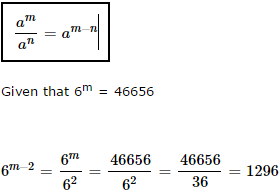Solution 2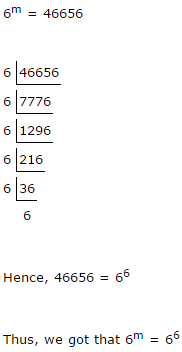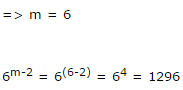QUESTION: 9

10222 ÷ 10220 = ?

Solution: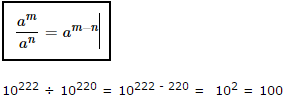QUESTION: 10

If m and n are whole numbers and mn = 196, what is the value of (m - 3)(n+1) ?

Solution:

mn = 196

We know that 142 = 196

Hence we can take m = 14 and n = 2

(m - 3)(n+1) = (14 - 3)(2+1) = 113 = 1331